Effect of turbulent atmosphere on the on-axis average intensity of Pearcey–Gaussian beam
Laboratory of Nuclear, Atomic, and Molecular Physics, Department of Physics, Faculty of SciencesChouaïb Doukkali University, P. O. Box 20, 24000 El Jadida, Morocco

† Corresponding author. E-mail: belafhal@gmail.com

Abstract
Abstract

The propagation characteristics of the Pearcey–Gaussian (PG) beam in turbulent atmosphere are investigated in this paper. The Pearcey beam is a new kind of paraxial beam, based on the Pearcey function of catastrophe theory, which describes diffraction about a cusp caustic. By using the extended Huygens–Fresnel integral formula in the paraxial approximation and the Rytov theory, an analytical expression of axial intensity for the considered beam family is derived. Some numerical results for PG beam propagating in atmospheric turbulence are given by studying the influences of some factors, including incident beam parameters and turbulence strengths.

PACS: 42.68.Bz;42.60.Jf
1. Introduction

Diffraction catastrophe theory is the theoretical foundation for generating accelerated beams such as Airy beams which are derived from fold diffraction catastrophe, Pearcey beams from cusp diffraction catastrophe, regular polygon beams from Thom’s elliptic umbilics,[3,4] and other accelerated beams that were recently introduced. Hence, based on the relation between diffraction integrals and catastrophe polynomials,[8,9] a general canonical catastrophe integral can be obtained. So, to realize a diffraction catastrophe, one of the methods is to use phase-only diffraction patterns. The key task of such a method is to produce an appropriate phase mask. Hence, the corresponding phase profile masks can be obtained on the basis of caustic characteristic diffraction pattern. For example, as is well known, 3/2 phase only pattern can generate Airy beams, observed experimentally for the first time by Siviloglou et al., and the quartic polynomial exponent phase can generate Pearcey beams. These last new kinds of paraxial beams were formulated by Ren et al., based on the Pearcey function of catastrophe theory.

The propagating form of the Pearcey beam has several noteworthy properties, some of which are reminiscent of not only Airy beams, but also Gaussian and Bessel beams. Like the Bessel beam and Airy beam, a pure Pearcey beam has infinite energy, but can be made finite by modulating the Pearcey function with a Gaussian one in real space, which does not significantly change the beam properties. Earlier, it was shown that the Pearcey beams are auto-focusing and self-healing after being distorted by obstacles. In a recent paper, a virtual source was proposed to generate the Pearcey beams. In Ref.  Pearcey beams were generalized into half Pearcey beams and it is shown that such beams, which are form-invariant,[12,15,16] propagate with acceleration and auto-focusing.

Recently, a lot of attention was paid to studying the propagation of some laser beams in a turbulent atmosphere because of their large applications in optical communications, imaging systems and remote sensing. As is well known, when a laser beam propagates in the atmosphere, it produces random variations in amplitude and phase of the electric field caused by random changes of the refractive index. In our research group, the propagations of Li’s flat-topped beams, Bessel-modulated Gaussian beams, Superposition of Kummer beams, truncated modified Bessel modulated Gaussian beams with quadrature radial dependence (MQBG), and Li’s flattened Gaussian beams in turbulent atmosphere were investigated. In Ref. , the propagation of PG beams in free space has been given. But to our knowledge, the propagation of PG beams in turbulent atmosphere has not been studied elsewhere.

In this paper, our interest is to study the propagation characteristics of PG beams through a turbulent atmosphere. The rest of this paper is organized as follows. In Section 2, an analytical expression of the average intensity of the considered beams is given based on the extended Huygens–Fresnel integral formula in the paraxial approximation and on the Rytov theory. An approximate analytical axial intensity distribution is derived in Section 3. We present some numerical simulations in Section 4. Finally, a conclusion is drawn from the present work in Section 5.

2. Average intensity of PG beams in turbulent atmosphere

In this section, we give the expression of the one-dimensional average intensity of the PG beams after propagating in turbulent atmosphere. The Pearcey function is defined by an integral representation[38,39] as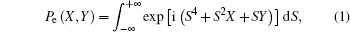where X and Y are dimensionless variables transverse to propagation in the z direction. In real space, the Pearcey function is Pe(x/x0,y/y0), where x0 and y0 are the specified scaling lengths along axes x and y, respectively.

The field distribution of the PG beams in the Cartesian coordinates (x1,y1, z) at the plane z = 0 is given by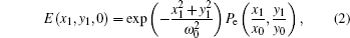where ω0 is the waist of the Gaussian party of the beam.

We illustrate in Figs. 14 the two-dimensional intensity of PG beams at the input plane (z = 0).

 Figure Option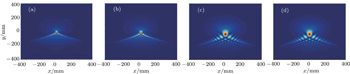Fig. 1. Incident transverse intensities of PG beams in source plane (z = 0) for ω0 = 0.5 mm and λ = 1550 nm with (a) x0 = y0 = 1 × 10−2 mm, (b) x0 = y0 = 1.2 × 10−2 mm, (c) x0 = y0 = 1.6 × 10−2 mm, and (d) x0 = y0 = 3 × 10−2 mm, respectively.
 Figure Option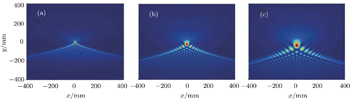Fig. 2. Incident transverse intensities of PG beams in source plane (z = 0) for ω0 = 1 mm and λ = 1550 nm with (a) x0 = y0 = 1 × 10−2 mm, (b) x0 = y0 = 2 × 10−2 mm, and (c) x0 = y0 = 3 × 10−2 mm, respectively.
 Figure Option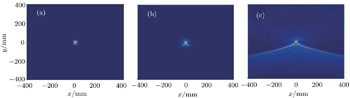Fig. 3. Incident transverse intensities of PG beams in source plane (z = 0) for λ = 1550 nm, x0 = y0 = 1.064 × 10−2 mm with (a) ω0 = 5 × 10−2 mm, and (b) ω0 = 0.1 mm, and (c) ω0 = 3 mm, respectively.
 Figure Option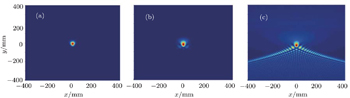Fig. 4. Incident transverse intensities of PG beams in source plane (z = 0) for λ = 1550 nm, x0 = y0 = 2 × 10−2 mm with (a) ω0 = 5 × 10−2 mm, (b) ω0 = 0.1 mm, and (c) ω0 = 3 mm, respectively.

Based on the extended Huygens–Fresnel integral formula in the paraxial approximation and on the Rytov theory, the expression of the electric field of the PG beams after propagating in a turbulent atmosphere is written as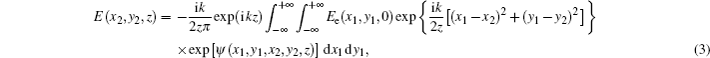where k = 2π/λ is the wavenumber, λ is the wavelength, z is the propagation distance, and ψ(x1,y1,x2,y2,z) represents the random part of the complex phase of a spherical wave propagating from the input plane to the output plane. A schematic diagram of a turbulent atmosphere optical system of the PG beams is illustrated in Fig. 5.

 Figure Option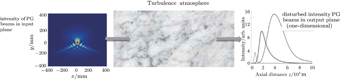Fig. 5. A schematic propagation of the PG beams in a turbulent atmosphere optical system.

In the following, we only consider a one-dimensional expression of the electric field of the PG beams at the z = 0 plane, which is expressed as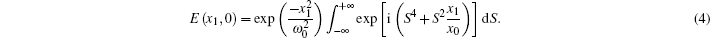Substituting Eq. (4) into Eq. (3), one obtains the expression of the average intensity at the output plane as follows: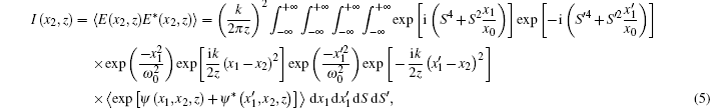where * and 〈 〉 denote the complex conjugate and ensemble average over the turbulent medium, respectively. For obtaining the last equation, we have used the following identity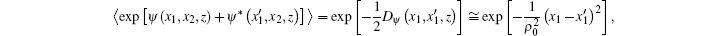where Dψ is the wave structure function of the random complex phase in the Rytov’s representation, and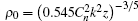is the coherence length of a spherical wave propagating in the turbulent medium, with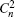being the refractive index structure constant indicating the turbulent strength.

3. Axial intensity distribution of PG beams in turbulent atmosphere

In this section, we derive the axial intensity distribution of PG beams in turbulent atmosphere using Eq. (5). Let x2 = 0, then one will obtain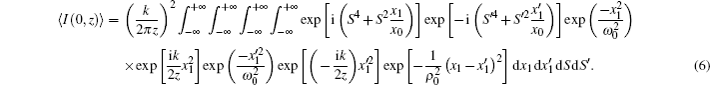To obtain the analytical expression of the axial intensity, the following integral formulas are used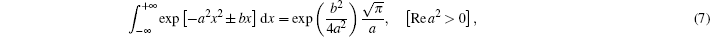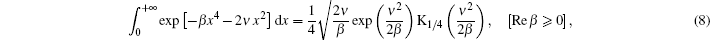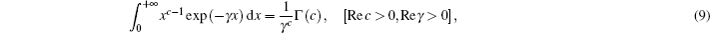where Γ and K1/4 are the Euler Gamma function and the modified Bessel function of the second kind, respectively.

Finally, after tedious calculations, the analytical expression of the axial intensity distribution of PG beams in turbulent atmosphere is written aswhere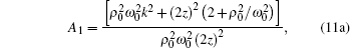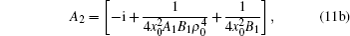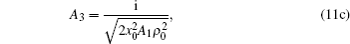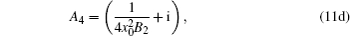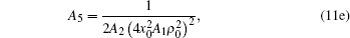with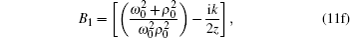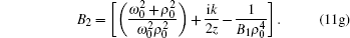Equation (10) is determined completely by Eqs. (11a)–(11g). This equation represents the analytical expression of the axial intensity, and it is the main result of the present work. In the following, we will use it for studying numerically the influences of the beam parameters, and the turbulent strength characterizing the turbulent atmosphere.

4. Numerical simulations

We illustrate in Fig. 6 the variations of on-axis average intensity with propagation distance z of PG beams for three values of turbulent strength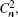two values of the specified scaling length x0 of the beam, λ = 632.8 nm and ω0 = 1 mm. The curves show that the intensity is equal to zero within the first several hundred meters of the near field when the turbulent strength increases and that for each value ofthe average intensity first increases, and then decreases with the increase of the propagation distance. We note that the axial intensity increases withincreasing and also with x0 decreasing. From these plots, we can also see that the position of the maximum depends on the turbulent strength i.e., the axial intensity vanishes quickly for the large values of turbulent strength. Moreover, the position of the maximum moves towards small value of the propagation distance when the specified scaling length x0 increases, which means that the Pearcey–Gaussian beam is affected by the turbulent atmosphere and the specified scaling length x0, i.e., the propagation is shorter when the atmosphere is very turbulent and x0 is greater.

 Figure Option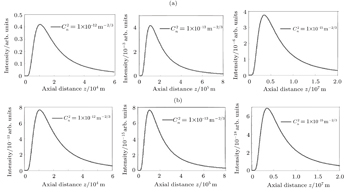Fig. 6. Plots of on-axis average intensity versus the propagation distance z of PG beam for (a) x0 = 2 × 10−2 mm and (b) x0 = 6 × 10−2 mm, three values of the turbulent strengths, λ = 632.8 nm, and ω0 = 1 mm, respectively.

To show how the beam wavelength affects the on-axis average intensity, we illustrate the variations of on-axis average intensity with propagation distance z in Fig. 7, for the chosen values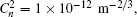x0 = 2 × 10−2 mm, and two values of the beam waist: ω0 = 1 mm and ω0 = 0.5 mm, respectively. We can see from this figure that the axial intensity is equal to zero beyond the first several kilometers and it increases with wavelength decreasing. We note from the illustrations that the position of the maximum depends on the wavelength and the axial intensity vanishes rapidly for the small values of λ. From these illustrations, we can see that the position of the maximum moves with propagation distance decreasing when the beam waist increases. Our numerical results show that the propagation in turbulent atmosphere of PG beam with smaller wavelength λ is shorter than that with larger wavelength.

 Figure Option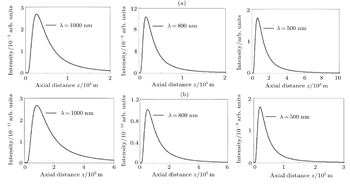Fig. 7. Plots of on-axis average intensity versus propagation distance z of PG beam for (a) ω0 = 1 mm and (b) ω0 = 0.5 mm, three values of λ,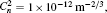and x0 = 2 × 10−2 mm, respectively.

Figure 8 shows the variations of on-axis average intensity with propagation distance z of PG beam for λ = 632.8 nm, x0 = 2 × 10−2 mm, and two values of turbulent strengthrespectively. For each value, three curves are plotted for different waist values. It is shown that the intensity profile keeps unchanged and the axial intensity is equal to zero beyond the first thousand meters in Fig. 8(a) and the first thousand kilometers in Fig. 8(b). From this figure, we also observe that the axial intensity increases with ω0 increasing and it vanishes very quickly for the large values of the beam waist. We note that the position of the maximum depends on the waist and moves with propagation distance decreasing as turbulent strength increases. This phenomenon can be explained physically by the fact that the PG beam with larger beam waist ω0 spreads more rapidly than with smaller beam waist propagating in turbulent atmosphere.

 Figure Option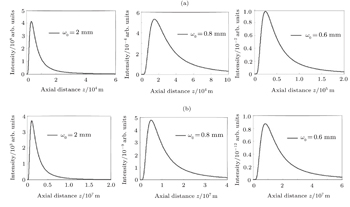Fig. 8. Plots of on-axis average intensity versus the propagation distance z of PG beams with three values of ω0, λ = 632.8 nm, x0 = 2 × 10−2 mm for (a)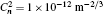and (b)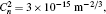respectively.
5. Conclusions

In this work, we derive an analytical expression of the axial intensity for PG beams, based on the extended Huygens–Fresnel integral formula in the paraxial approximation and on the Rytov theory, in turbulent atmosphere. Our numerical simulations shown that the PG beams with larger wavelength and smaller waist are less affected by the atmospheric turbulence, which is useful for applications in the field of optical communication for a long distance.

Reference
 1 Vallée OSoares M2004Airy functions and applications to physicsLondonImperial College Press 2 Nye J F J2003Opt. A: Pure Appl. Opt.5495 3 Barwick S2010Opt. Lett.354118 4 Barwick S2010Appl. Opt.496893 5 Zhang Y TZhang Z GCheng TZhang Q CWu X P2015Chin. Phys. Lett.3214205 6 Ren Z JLi X DFan C JXu Z Q2013Chin. Phys. Lett.30114208 7 Ren Z JLi X DJin H ZChen CWu J M2014Chin. Phys. B23084202 8 Nye J F2009J. Opt. A: Pure Appl. Opt.11065708 9 Berry M VBalazs N L1979Am. J. Phys.47264 10 Cottrell D MDavis J AHazard T M2009Opt. Lett.342634 11 Siviloglou G ABroky JDogariu AChristodoulides D N2007Phys. Rev. Lett.99213901 12 Ring J DLindberg JMourka AMazilu MDholakia KDennis M R2012Opt. Express2018955 13 Ren Z JYin CJin H JChen B2015J. Opt.17105608 14 Deng DChen CZhao XChen BPeng XZheng Y2014Opt. Lett.392703 15 Kovalev A AKotlyar V VZaskanov S GPorfirev A P2015J. Opt.17035604 16 Kovalev A AKotlyar V VZaskanov S G2015Proc. SPIE944894482 17 Wang L GZheng W W2009J. Opt. A11065703 18 Wen WChu XMa H2015Opt. Commun.336326 19 Eyyuboglu H THardalac F2008Opt. Laser Technol.40343 20 Eyyuboglu H T2008Opt. Laser Technol.40156 21 Cai YEyyuboglu HBaykal Y2008Opt. Commun.2815291 22 Zhou PLiu ZXu XChu X2009Opt. Laser Technol.41403 23 Liu XLiang CYuan YCai YEyyuboglu H T2013Opt. Laser Technol.45587 24 Chu X2011Opt. Lett.362701 25 Li Y QWu Z SWang M J2014Chin. Phys. B23064216 26 Li Y QWu Z SZhang Y YWang M J2014Chin. Phys. B23074202 27 Qian X MZhu W YRao R Z2012Chin. Phys. B21094202 28 Li Y QWu Z S2012Chin. Phys. B21054203 29 Andrews L CPhillips R LHopen C Y2001Laser beam scintillation with applicationsWashingtonSPIE Press201 30 Cang JZhang Y2010Optik.121239 31 Noriega-Manez R JGutiérrez-Vega J C2007Opt. Express1516328 32 Korotkova OGbur G2007Proc. SPIE645764570 33 Kinani AEz-zariy LChafiq ANebdi HBelafhal A2011Phys. Chem. News6124 34 Belafhal AHennani SEz-zariy LChafiq AKhouilid M2011Phys. Chem. News6236 35 Khannous FNebdi HBoustimi MKhouilid MBelafhal A2014Phys. Chem. News7383 36 Hennani SBarmaki SEz-zariy LNebdi HBelafhal A2013Phys. Chem. News6944 37 Khannous FBoustimi MNebdi HBelafhal A2014Phys. Chem. News7373 38 Berry M VHowls C J2012“Integrals with coalescing saddles,” http://dlmf.nist.gov/36.2 (Digital Library of Mathematical Functions, National Institute of Standards and Technology) 39 Pearcey T1946Phil. Mag. S.37311 40 Andrews LPhilips R1998Laser beams propagation through random mediaWashingtonSPIE Press57 41 Gradshteyn I SRyzhik I M1994Tables of Integrals, Series and ProductsNew YorkAcademic Press